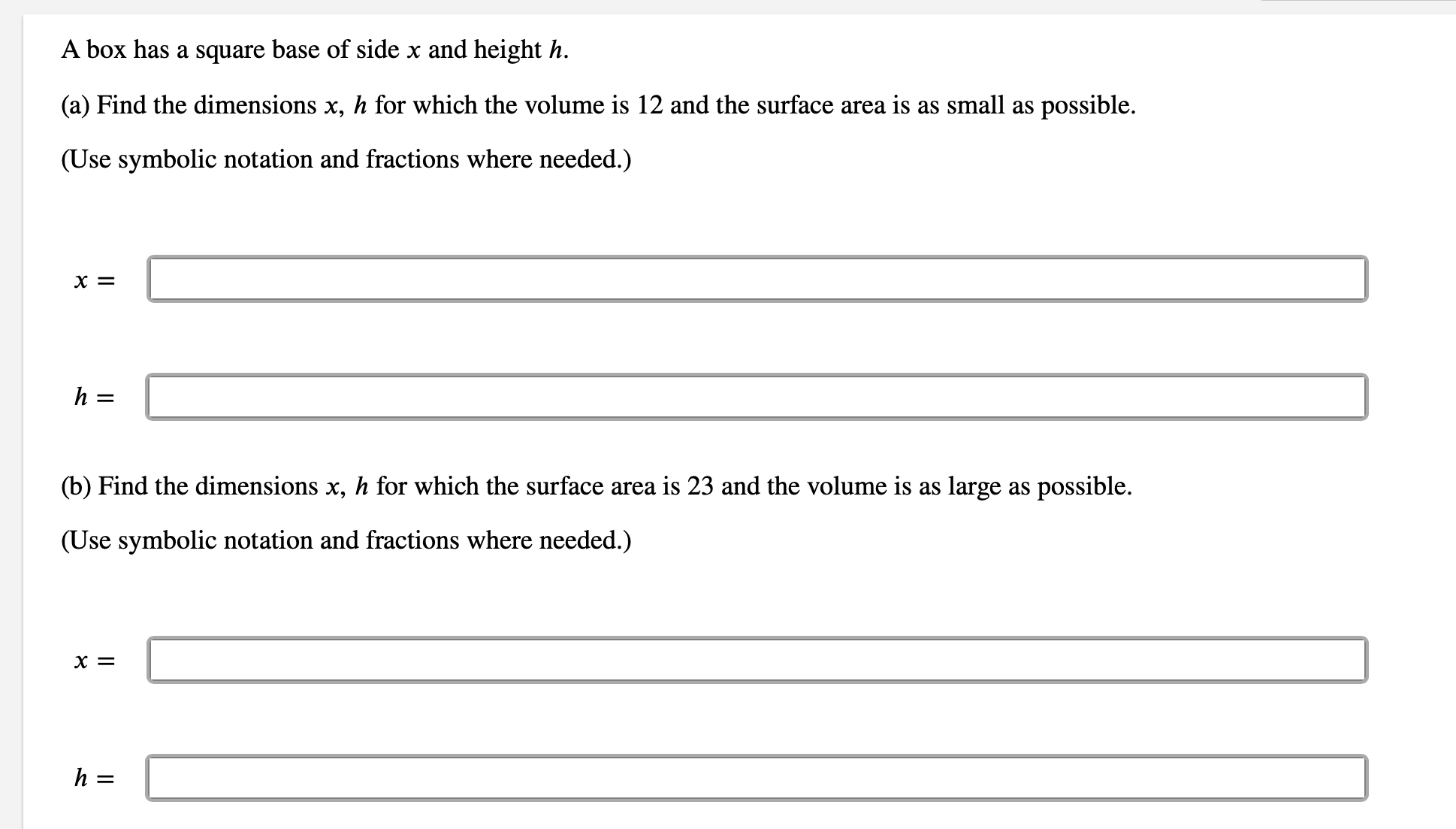# A box has a square base of side x and height h. (a) Find the dimensions x, h for which the volume is 12 and the surface area is as small as possible. (Use symbolic notation and fractions where needed.) X = h = (b) Find the dimensions x, h for which the surface area is 23 and the volume is as large as possible. (Use symbolic notation and fractions where needed.) X = h =

Questionhelp_outlineImage TranscriptioncloseA box has a square base of side x and height h. (a) Find the dimensions x, h for which the volume is 12 and the surface area is as small as possible. (Use symbolic notation and fractions where needed.) X = h = (b) Find the dimensions x, h for which the surface area is 23 and the volume is as large as possible. (Use symbolic notation and fractions where needed.) X = h = fullscreen

### Want to see this answer and more?

Experts are waiting 24/7 to provide step-by-step solutions in as fast as 30 minutes!*

*Response times vary by subject and question complexity. Median response time is 34 minutes and may be longer for new subjects.
Tagged in
MathCalculus

### Other